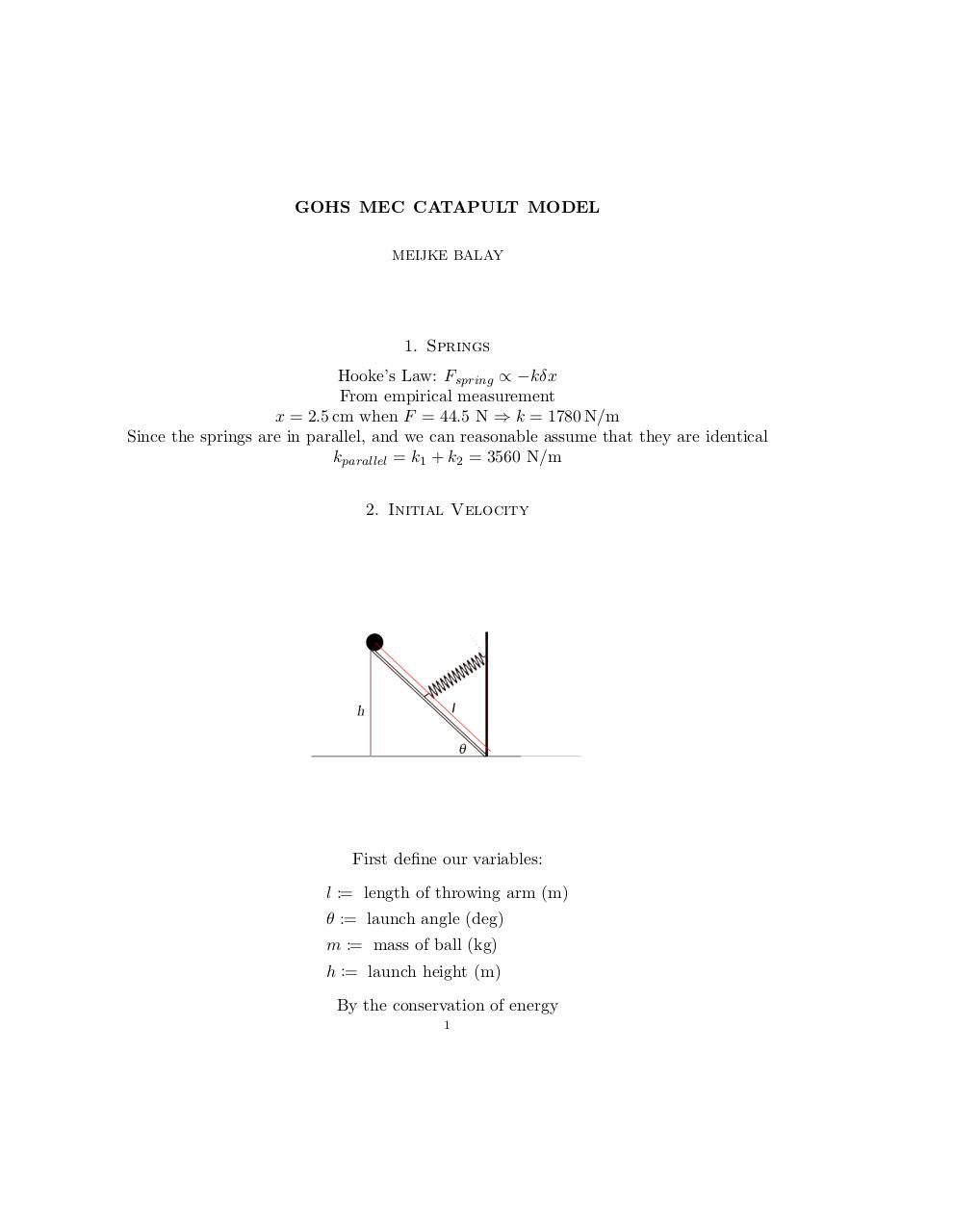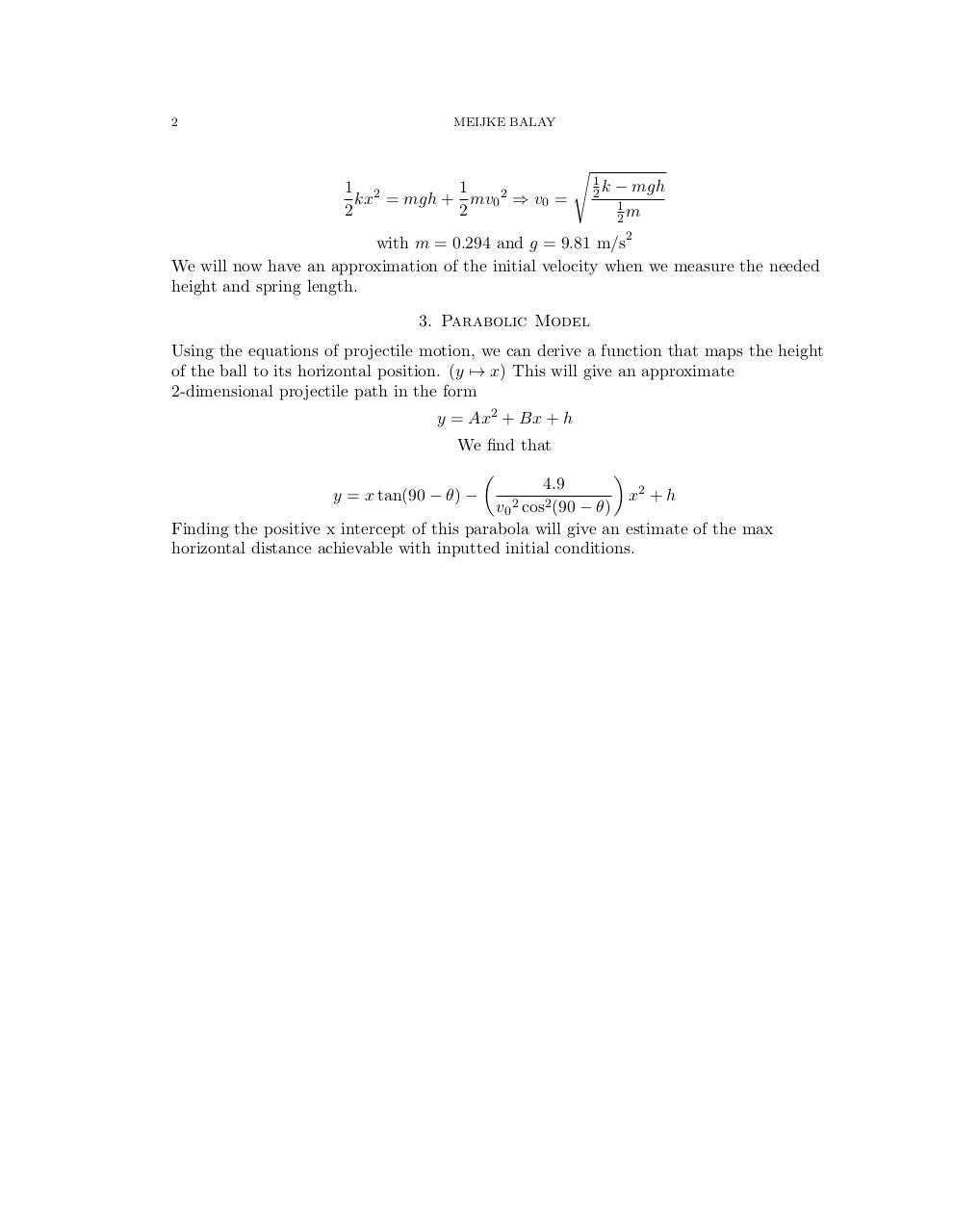# PDF Archive

Easily share your PDF documents with your contacts, on the Web and Social Networks.

## MEC Catapult Model (Draft) .pdf

Original filename: MEC Catapult Model (Draft).pdf

This PDF 1.5 document has been generated by TeX / pdfTeX-1.40.16, and has been sent on pdf-archive.com on 13/03/2016 at 02:23, from IP address 173.55.x.x. The current document download page has been viewed 366 times.
File size: 113 KB (2 pages).
Privacy: public file### Document preview

GOHS MEC CATAPULT MODEL
MEIJKE BALAY

1. Springs
Hooke’s Law: Fspring ∝ −kδx
From empirical measurement
x = 2.5 cm when F = 44.5 N ⇒ k = 1780 N/m
Since the springs are in parallel, and we can reasonable assume that they are identical
kparallel = k1 + k2 = 3560 N/m
2. Initial Velocity

First define our variables:
l := length of throwing arm (m)
θ := launch angle (deg)
m := mass of ball (kg)
h := launch height (m)
By the conservation of energy
1

2

MEIJKE BALAY

1 2
1
kx = mgh + mv0 2 ⇒ v0 =
2
2

s

1
2k

− mgh
1
2m

with m = 0.294 and g = 9.81 m/s2
We will now have an approximation of the initial velocity when we measure the needed
height and spring length.
3. Parabolic Model
Using the equations of projectile motion, we can derive a function that maps the height
of the ball to its horizontal position. (y 7→ x) This will give an approximate
2-dimensional projectile path in the form
y = Ax2 + Bx + h
We find that

4.9
x2 + h
y = x tan(90 − θ) −
v0 2 cos2 (90 − θ)
Finding the positive x intercept of this parabola will give an estimate of the max
horizontal distance achievable with inputted initial conditions.